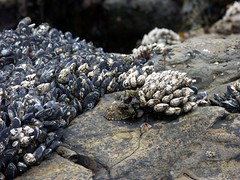Competition is considered by many ecologists to be a major structuring factor in communities. It is a notoriously difficult thing to identify, classify and measure in the field and has been, in my opinion, an inspiration for some of the more elegant field studies. There is no doubt that species compete for resources in nature, but more elusive are answers to how much that competition matters to the stability of a species population, and the community as a whole, and what role competition might play on longer, evolutionary timescales. Typically, when we wish to measure competition, we require a few pieces of basic data, such as population sizes, interaction strengths and frequencies with the resource(s) being competed for, age structuring and so on. How can we go about doing this with complex food webs lacking these data? As usual, my answer is that you cannot, simply because of a lack of data. Nevertheless, I think that complex food webs do have something to say about competition, as long as one realizes that there is a trade-off between details of microscopic interspecific interactions and grabbing a macroscopic view of the community. Recently I’ve been mulling over appropriate ways to do this, and here are some ideas. I will preface them by saying that the interest stems from examining the potential impact of an invasive species as a competing consumer.

Let us begin with a (asymmetric) binary adjacency matrix, A, whose elements$a_{ij}$ indicate whether species i preys on species j. The question is, what is the interaction between two consumer species, i and m. My first step is to simply count the number of prey shared between i and m, measured as the Hamming distance between the$i^{\text{th}}$ and$m^{\text{th}}$ rows; let’s designate that$H_{im}$ ($=H_{mi}$). We can refine our view a bit by asking what fraction of a species’ prey is represented by that overlap, which is simply$\frac{k_{i}-H_{im}}{k_{i}}$
where$k_{i}$ is the in-degree, or number of prey for species i in the food web network. You can think of this as the potential impact of species m on i. This is not quite satisfactory though, because$k_{i}$ and$k_{m}$ may be vastly different. For example, in our Caribbean coral reef food webs, many reef foraging piscivores (fish eaters) are specialists, preying mostly on maybe six other species, with those prey also being part of the repertoire of more generalist piscivores such as carcharhinid sharks who also forage on the reef and have k in the range of 70-80. It would be difficult to conceive of two such consumers as being strong competitors if the interactions of the generalist are distributed broadly over its prey. I therefore assume, in the absence of data on population densities, interaction strengths and functional responses of predators to prey, that this network measure of competitive interaction will be a function of both prey overlap (H) and consumer dietary breadth (k). There will be a trend of increasing pairwise strength of competitive interaction from generalist-generalist to generalist-specialist to specialist-specialist.

We can now extend our formulation in the following manner. First, count the number of prey shared between the consumers,$I_{im}$. Then weight the interaction strength between m and its prey uniformly according to$k_{m}$ (ala CEG). The total interaction strength is$\frac{I_{im}}{k_{m}}$
which is also the fraction of i’s prey that is being affected by m’s predation. The unaffected fraction, standardized to i’s dietary breadth is$\frac{1}{k_{i}}\left (k_{i} - \frac{I_{im}}{k_{m}}\right )$
yielding a standardized impact of$\frac{I_{im}}{k_{i}k_{m}}$
Note that this index is symmetric for i and m, i.e., it is the SAME for both species.

As a worked example, consider four species, A, B, C and D, with k’s of 60, 70, 2 and 2 respectively. The overlap of resources are: AB-35, AC-2, CD-1. The competitive indices are$\alpha_{AB} = 0.0083$$\alpha_{AC} = 0.017$
and$\alpha_{CD} = 0.25$
I use$\alpha$ in keeping with a conventional symbol for competitive interaction, but again point out that this is a very unparameterized measure compared to what is normally considered for use in Lotka-Volterra-type models or as measured empirically. You’ll notice that the values increase as the specialization of the interactors increases. It would be nice to scale these to a unit maximum to facilitate comparison, but I haven’t done that yet.

In a follow-up post I’ll provide some worked examples of all the above using real species from a real coral reef food web!